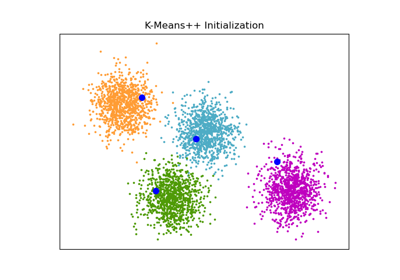# sklearn.cluster.kmeans_plusplus¶

sklearn.cluster.kmeans_plusplus(X, n_clusters, *, x_squared_norms=None, random_state=None, n_local_trials=None)[source]

Init n_clusters seeds according to k-means++.

New in version 0.24.

Parameters:
X{array-like, sparse matrix} of shape (n_samples, n_features)

The data to pick seeds from.

n_clustersint

The number of centroids to initialize.

x_squared_normsarray-like of shape (n_samples,), default=None

Squared Euclidean norm of each data point.

random_stateint or RandomState instance, default=None

Determines random number generation for centroid initialization. Pass an int for reproducible output across multiple function calls. See Glossary.

n_local_trialsint, default=None

The number of seeding trials for each center (except the first), of which the one reducing inertia the most is greedily chosen. Set to None to make the number of trials depend logarithmically on the number of seeds (2+log(k)).

Returns:
centersndarray of shape (n_clusters, n_features)

The initial centers for k-means.

indicesndarray of shape (n_clusters,)

The index location of the chosen centers in the data array X. For a given index and center, X[index] = center.

Notes

Selects initial cluster centers for k-mean clustering in a smart way to speed up convergence. see: Arthur, D. and Vassilvitskii, S. “k-means++: the advantages of careful seeding”. ACM-SIAM symposium on Discrete algorithms. 2007

Examples

>>> from sklearn.cluster import kmeans_plusplus
>>> import numpy as np
>>> X = np.array([[1, 2], [1, 4], [1, 0],
...               [10, 2], [10, 4], [10, 0]])
>>> centers, indices = kmeans_plusplus(X, n_clusters=2, random_state=0)
>>> centers
array([[10,  4],
[ 1,  0]])
>>> indices
array([4, 2])


## Examples using sklearn.cluster.kmeans_plusplus¶An example of K-Means++ initialization

An example of K-Means++ initialization